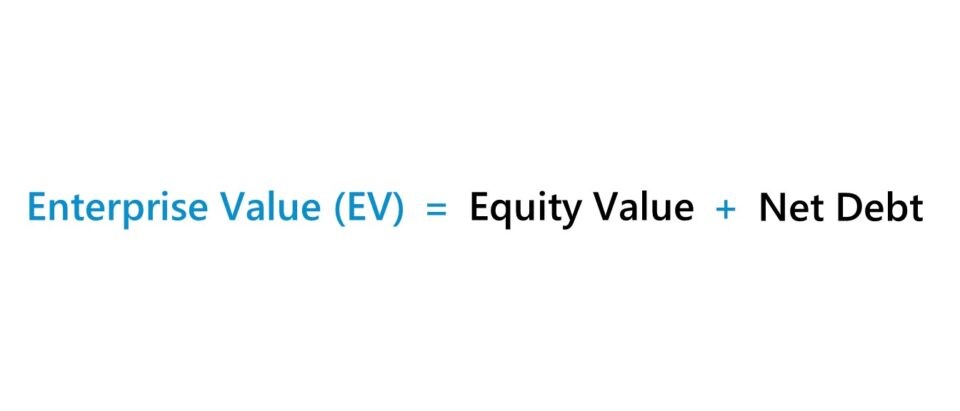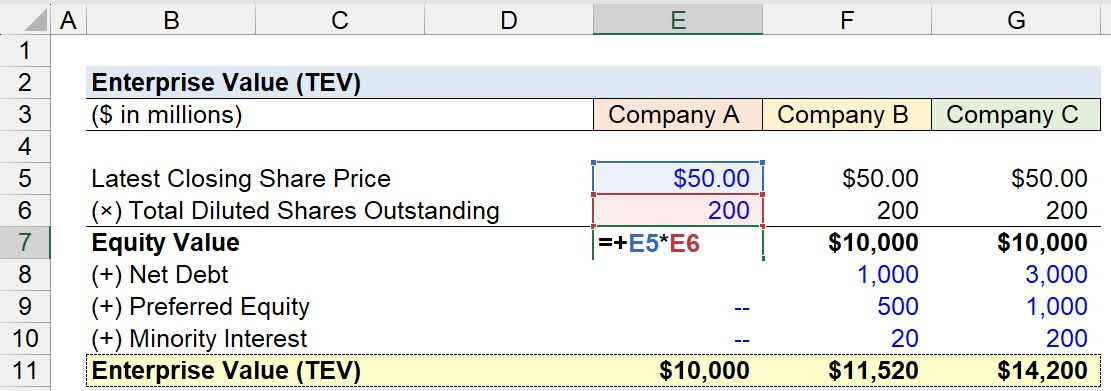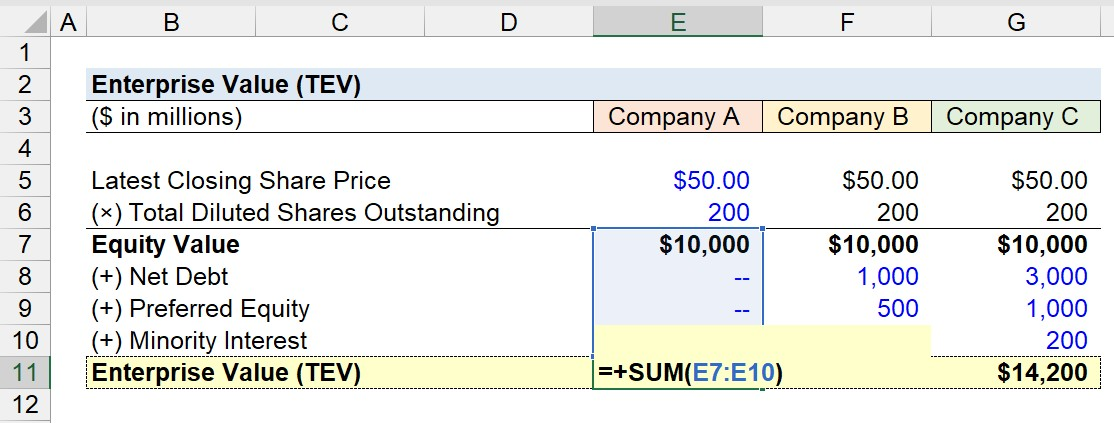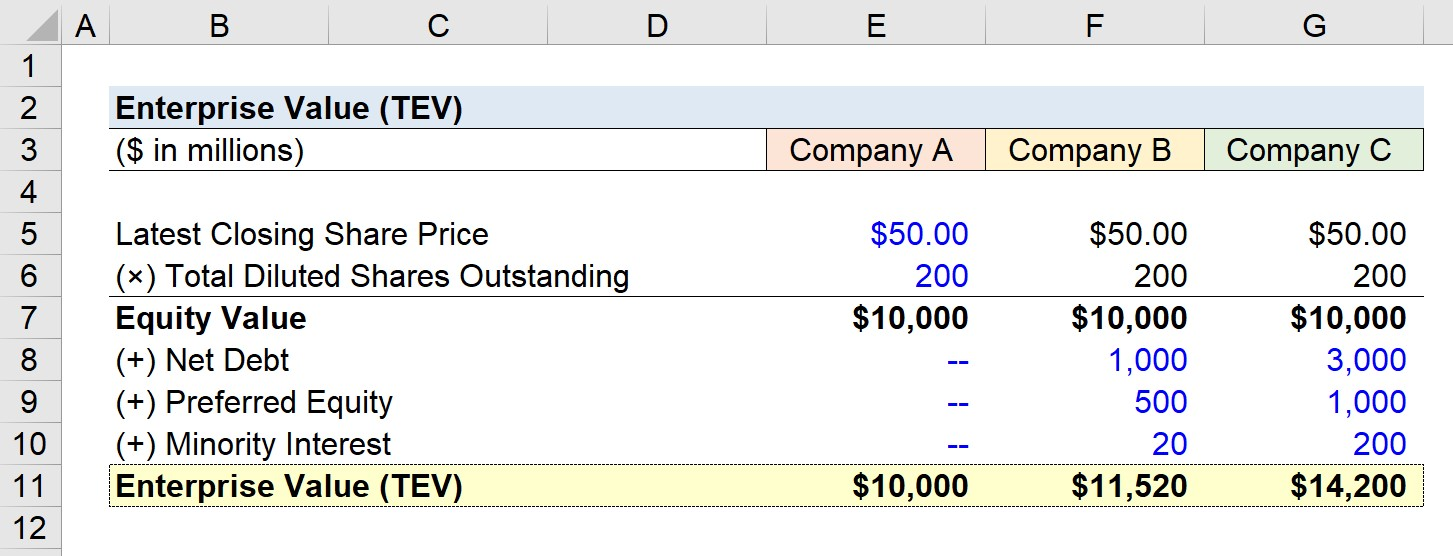Welcome to Wall Street Prep! Use code at checkout for 15% off.# Enterprise Value (TEV)

Guide to Understanding Enterprise Value (TEV)## How to Calculate Enterprise Value?

The enterprise value measures the value of a company’s operations to all stakeholders, including equity and debt capital providers. Hence, the enterprise value is considered to be a capital structure-neutral metric.

Unlike the equity value metric – otherwise referred to as market capitalization (or “market cap”) – the enterprise value is unaffected by the discretionary financing decisions of the management team.

In effect, enterprise value reflects the value of the core operations of a business, irrespective of its capitalization (i.e. financing mix), which facilitates more accurate comparisons between companies due to being independent of their different capital structures.

Conceptually, enterprise value quantifies how much the core operating business is worth (i.e. operating assets minus operating liabilities) to all stakeholders.

For instance, if a company’s debt-to-equity ratio were to increase after raising more debt capital, its enterprise value should theoretically remain unchanged – despite some minor impacts on the company’s financial statements.

In order to calculate the enterprise value of a company, you start by taking the company’s equity value and then add net debt, preferred stock, and minority interest (i.e. non-controlling interest).

1. Calculate Equity Value → Our starting point, the equity value (i.e. the “market cap”), represents the value of the entire company to only one group of capital providers, which is the common shareholders.
2. Add Net Debt → From there, while not explicitly broken out, the net debt calculation subtracts any non-operating assets – more specifically, cash and cash equivalents (e.g. marketable securities, commercial paper, short-term investments) – from the total amount of debt and any interest-bearing instruments.
3. Add Value of Other Equity Related Claims → In the subsequent step, we’ll add the liabilities and equity items representative of the stakes held by all other investor groups, e.g. minority interest and preferred equity.

## Enterprise Value Formula

The formula to calculate the enterprise value of a company is as follows.

Enterprise Value (TEV) = Equity Value + Net Debt + Preferred Stock + Minority Interest

Where:

Net Debt = Total Debt Cash and Cash Equivalents

So, why is cash subtracted from enterprise value?

The rationale behind incorporating net debt rather than gross debt is that the cash sitting on a company’s balance sheet could hypothetically be used to pay down outstanding debt if deemed necessary.

## Enterprise Value vs. Equity Value: What is the Difference?

To calculate equity value from TEV, the reverse calculation should be performed, in which net debt is first subtracted, and then all non-common equity claims are deducted (i.e. removing the claims of preferred shareholders).

Equity Value = Enterprise Value Net Debt Preferred Stock Minority Interest

The image below illustrates the relationship between enterprise value and equity value (i.e. “market cap”).

## What are Examples of Enterprise Value Multiples?

Because of the fact enterprise value is capital structure neutral, the metric is the most widely used measure of value in relative valuation, in which TEV-based multiples are used far more frequently in practice than equity value multiples – especially in the context of M&A.

The most common enterprise value-based multiples are the following:

TEV/EBITDA = Enterprise Value ÷ EBITDA
TEV/EBIT = Enterprise Value ÷ EBIT
TEV/Revenue = Enterprise Value ÷ Revenue

Like the numerator, the denominator (e.g. EBITDA, EBIT) also represents all stakeholders in a company, as opposed to a single stakeholder group like in the case of net income – whereas TEV/Net Income is not a viable valuation multiple due to the mismatch in the applicable investor groups.

Along the same lines, the enterprise value corresponds to the weighted average cost of capital (WACC), which is the weighted discount rate (i.e. hurdle rate) with all capital providers in mind.

In contrast, the cost of equity is the appropriate discount rate when calculating the equity value.

The key takeaway is that the equity value of a company is the residual value left for common shareholders, whereas the enterprise value represents all capital contributors.

If a company has a negative TEV, that means the company has a net cash balance (i.e. the total cash minus total debt) that exceeds its equity value. While a company with a negative enterprise value is an irregular occurrence, it can occasionally occur.

## How to Calculate Enterprise Value Using Valuation Multiple?

One of the most common valuation multiples is the TEV/EBITDA multiple, which compares the total value of a company’s operations (EV) relative to its EBITDA.

With that said, EBITDA in valuation multiples is particularly useful for capital-intensive companies, where a significant amount of capital is allocated to the purchase of fixed assets.

Given how D&A is a direct function of a company’s capital expenditures, companies with asset-heavy business models are susceptible to periodic fluctuations in performance that can skew GAAP financial results.

The TEV/EBITDA multiple answers the following question, “For each dollar of EBITDA generated, how much are the company’s investors currently willing to pay?”

In order to compute a company’s enterprise value (TEV) using the TEV/EBITDA multiple, the company’s EBITDA is multiplied by the EBITDA multiple to arrive at the implied valuation.

TEV/EBITDA Multiple = Enterprise Value ÷ EBITDA
Enterprise Value (TEV) = EBITDA × TEV/EBITDA Multiple

## Enterprise Value Calculator

We’ll now move on to a modeling exercise, which you can access by filling out the form below.Submitting...

## 1. Equity Value Calculation Example

Suppose we’re looking at three different companies with identical share prices as well as share counts.

With those two assumptions stated, we can calculate that the equity value of all three companies is \$10 billion.

• Equity Value = \$50.00 × 200 million = \$10 billion## 2. Capital Structure Assumptions

In the prior step, we calculated the equity value, so we now just need the remaining assumptions to calculate the enterprise value of each company.

Company A Financials

• Net Debt = \$0m
• Preferred Equity = \$0m
• Minority Interest = \$0m

Company B Financials

• Net Debt = \$1bn
• Preferred Equity = \$500m
• Minority Interest = \$20m

Company C Financials

• Net Debt = \$3bn
• Preferred Equity = \$1bn
• Minority Interest = \$200m

Given the assumptions above, the pattern to note is how the company’s capital structure becomes increasingly more complex from Company A to Company C.

## 3. Enterprise Value Calculation Example

In the case of Company A, since all three assumptions regarding the non-common equity claims are zero, the enterprise value is equivalent to the equity value, i.e. Company A is an all-equity firm.

With all the necessary data listed, we can input each assumption into our model and calculate the enterprise value for each company, as shown below.The implied enterprise value for the three companies is as follows:

• TEV, Company A = \$10 billion
• TEV, Company B = \$11.5 billion
• TEV, Company C = \$14.2 billion

Our three hypothetical companies, despite having the same equity value, have very different operating values (i.e. enterprise values).

More specifically, the value of Company C’s core operating business is \$4.2bn greater than Company A’s.

## 4. Enterprise Value Calculation Analysis

Compared to the equity value, the enterprise value (EV) is closer to the real value of a company since the valuation metric accounts for all ownership stakes rather than just that of equity owners.

At this point, a common misunderstanding occurs: Students will look at the spreadsheet above and may ask themselves: “Why on earth does adding debt or preferred equity increase a company’s enterprise value?”

Well, the short answer is that the addition of debt or preferred equity does not increase enterprise value, contrary to a frequent misconception.

By raising capital via debt financing, the company also brings cash onto the books, meaning that the net debt remains the same if all that a company has done is take on more debt.Step-by-Step Online Course

### Everything You Need To Master Financial Modeling

Enroll in The Premium Package: Learn Financial Statement Modeling, DCF, M&A, LBO and Comps. The same training program used at top investment banks.Inline FeedbacksLearn Financial Modeling Online

Everything you need to master financial and valuation modeling: 3-Statement Modeling, DCF, Comps, M&A and LBO.

X

The Wall Street Prep Quicklesson Series

7 Free Financial Modeling Lessons

Get instant access to video lessons taught by experienced investment bankers. Learn financial statement modeling, DCF, M&A, LBO, Comps and Excel shortcuts.